# GRE Quantitative Comparison Geometry Practice ProblemsHere is a batch of 7 practice QC questions.  Explanations will come at the end of the article.

Note: all quantitative comparison questions have the same four answer choices.  I didn’t copy the answer choices for each question in this post, so please note the answer choices below:

a. Quantity A is greater.

b. Quantity B is greater.

c. The two quantities are equal.

d. The relationship cannot be determined from the information given.1) In the diagram, AC = 6.  CE = 12, DF = 4, and AB is parallel to DE.2)3) In the diagram, JL = 4 and JK = 6.4) In the diagram, O is the center of the circle, and AB is a diameter.  Region J is the area between chord AC and the arc of the circle.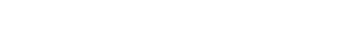5) In the diagram, triangle MNP is equilateral.6) In the diagram, JKLM is a square. Point S is the midpoint of KL, and point T is the center of the square.  Point O is on segment ST, and is the center of the circle, which passes through both K and L.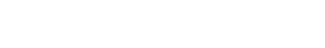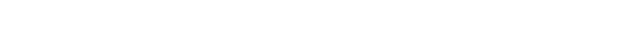7)## Geometry on the GRE QC

As you may appreciate, there’s no guarantee that any geometry diagram on the entire GRE Quant section is drawn to scale.  In fact, most diagrams that appear are specifically designed to hoodwink you in the most viciously deceptive way imaginable.  For example, if the GRE gives thiswith no further explanation or specification, the naïve gullible GRE test taker will think, “OK, an equilateral triangle,” whereas in fact, it could be any of these:Never be duped by believing a diagram.  In particular, one large group of GRE QC Geometry questions gives you diagrams that appear a certain way, but which leaves some ambiguity open, and your job is to spot the ambiguity, the different geometric possibilities, and not be sucked in by trusting the misleading diagram.  Some of the questions above are this sort.

Another batch of GRE QC Geometry questions specific everything in detail, and your job is to perform some kind of calculation, a length or angle or area, and compare it to something.

At the very least, it’s important to recognize, when looking at a GRE QC Geometry question, whether it’s the ambiguous-diagram kind or the everything-specified kind.  The former case has a high likelihood of having (D) as an answer!

## Summary

If the foregoing discussion gave you some insights into the question, you may want to go back and give them a second glance.  Please let us know about your experience with GRE Geometry questions in the comment sections below.## Practice problem explanations

1) The triangles CBA and CDE are similar, so all corresponding lengths are proportional.  Each length in CBA is 1/2 as big as the corresponding length in CDE.  Think about DF, the altitude in CDE, and the length to which it would correspond in CBA: an altitude from vertex B to the base AC.

Call that h.  Since DF = 4, h = 2.

Now, for CBA, we have an altitude h = 2 and a base AC = 6.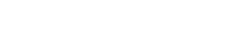2) Everything here is fixed and specified.  Triangle ABC is a right triangle, because the longest side is the diameter. See this GMAT blog post for details.  In right triangle ABC, AB = 4 is the hypotenuse.  Notice, this is NOT a 3-4-5 triangle, because the hypotenuse is 4.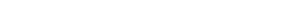We could take the square root to find the length of AC, but we don’t need to.  AC is the side of the square, so AC squared is the area of the square.  The square has an area of 7.

3) This is a deceptive diagram.  The angle at L appears to be right angle, but absolutely nothing in the diagram or the text guarantees that it is a right angle.  The triangle could be:On the left, the triangle has been “crushed” close to flat, and it could be crushed even further, to an area of almost zero.  Certainty the area could be less than 11.   By contrast, if JK and JL are perpendicular, then the area would be A = 0.5bh = (0.5)(4)(6) = 12, which is more than 11.  Depending on the diagram, it could go either way.

4) We know AB is a diameter, and we know that angle ACB is 90°.  All of that we know.  We don’t know the position of point C.  In the diagram given, point C is positioned so that the two areas look approximately equal.  By moving point C around, we could radically change the situation. For example, we could move C toward B, making Region J much larger.Or we could move point C toward the other side, closer to point A, in which case the triangle would be much bigger and Region J much smaller.Since we can vary point C to produce different relationship, we cannot produce a fixed answer.

5) This is a hard problem, but we can do it entirely without touching a calculator.

The first step is to draw the altitudes from each vertex of the equilateral triangle to the midpoint of the opposite side.  This will subdivide the equilateral triangle into six congruent 30-60-90 triangles.  For more details on these triangles, see this GMAT blog.  It’s very handy to remember that any equilateral triangle can be subdivided this way.Notice that OP, OM, and ON are all radii of the circle.  Let’s just say for convenience that r = 2, so OPO = OM = ON = 2.  Look at 30-60-90 triangle TOP.  The hypotenuse is OP = 2, so the side opposite the 30° angle is half of this, OT = 1.  The remaining side, opposite the 60°, must have a length of: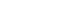The area of triangle TOP would be: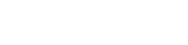Six of these triangles make up the entire equilateral triangle, so that’s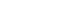That’s the area of the entire equilateral triangle.  Meanwhile, the circle has a radius of r = 2, so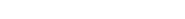Now the shaded area is the area of the circle minus the area of the equilateral, so the area of the shaded region is: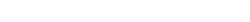Now, we have numbers for the areas of both regions:Whatever the square-root of 3 equals, it is clearly less than 2, so Quantity A is less than 2, and Quantity B is slightly more than 2.

6) Notice that the one thing not fixed in the problem is the position of point O on the segment ST.  It could be toward the top or bottom of that segment.  Think about the implications.

If we move point O toward the top of the circle, so that it is very close to S, then we get relative small circles.In the limit in which O was at S, the circle would be one whose diameter would equal the side of the square: such a circle could fit entirely inside the square, simply touching each side at its midpoint.  Such a circle clearly would have much less area than the square.

On the other hand, we could move point O down toward point T, the center of the square.  This produces relatively large circles.In the limit in which O was at T, the circle would neatly contain the square, passing through the four corners.  Such a circle clearly has more area than the square.

Because we can choose a position for point O that allows for a relationship either way, we can determine no conclusive relationship between the quantities.7) This is a hard question.  Let’s approach it this way.  Sometimes it is very helpful to introduce a variable into a geometric problem.  I am going to say that AC = x and BC = 1.  Notice that AB = x + 1.  Then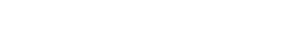Now, what happens if x = 3?  Notice that: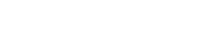Clearly, they are not equal, as they should be.  What happens if we make x bigger?  Well, then AC/BC = x simply becomes bigger, but AB/BC becomes smaller, so they wouldn’t get closer to being equal.  In fact, they would be getting further away from each other.

What happens if x get smaller?  Well, then AC/BC = x clearly gets smaller, and the fraction 1/x gets bigger when its denominator gets smaller, so AB/BC would get bigger.  In that case, they would be moving toward each other: the bigger one getting smaller, and the smaller one getting bigger.  That indicates they could become equal if we move x in that direction, smaller than 3.  In other words, x must be smaller than 3.

Right there, that’s enough to determine that the answer to the QC question is (B).

If you are interested, notice that if x = 2, then: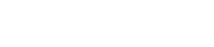They’re closer, but not equal.  If x = 1, then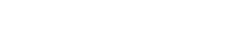Here, they have shot past each other.  Now, the little one is too big, and the big one is too little!   We overshot.  This tells us the correct value of x is between 1 and 2.

In fact, a few students may recognize that the diagram & equation above is the definition of the Golden Ratio, and in fact, this is the correct value of x.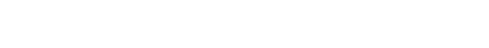If you happened to be familiar with the Golden Ratio, that would have been a shortcut on this particular problem, but in general, you don’t need to know anything about the Golden Ratio for the GRE.  The Golden Ratio plays a big role in sacred geometry and all kinds of esoteric wisdom (Egyptian temples, the Parthenon, Leonardo’s paintings, the Gothic Cathedrals, etc.), so you may find it interesting, but all this is well beyond anything relevant to success on the GRE!! 🙂

For even more GRE math practice, check out our GRE math questions with answers and explanations!

## Author

•Mike served as a GMAT Expert at Magoosh, helping create hundreds of lesson videos and practice questions to help guide GMAT students to success. He was also featured as "member of the month" for over two years at GMAT Club. Mike holds an A.B. in Physics (graduating magna cum laude) and an M.T.S. in Religions of the World, both from Harvard. Beyond standardized testing, Mike has over 20 years of both private and public high school teaching experience specializing in math and physics. In his free time, Mike likes smashing foosballs into orbit, and despite having no obvious cranial deficiency, he insists on rooting for the NY Mets. Learn more about the GMAT through Mike's Youtube video explanations and resources like What is a Good GMAT Score? and the GMAT Diagnostic Test.